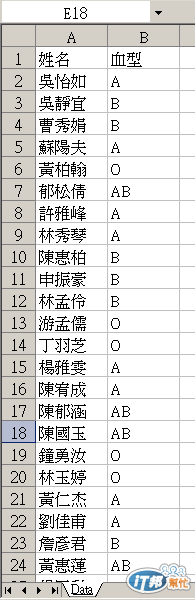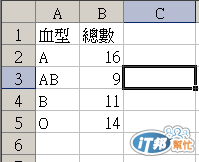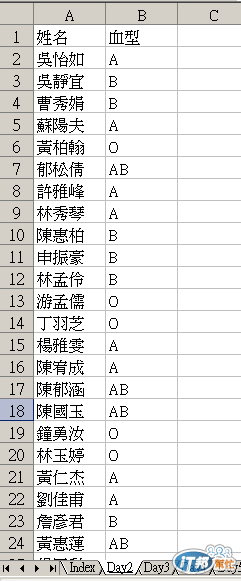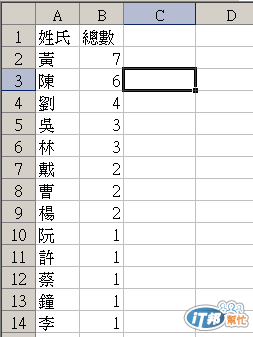#DAY 28
3

## Excel VBA 的眉眉角角Day28: SQL運用

``````Public Function SQL(strCommandText As String, Optional strXLSFileName As String, Optional strSheetName As String, Optional bnGotoSheet As Boolean = True, Optional bnDelSht As Boolean = True, Optional iRow As Integer = 1, Optional iCol As Integer = 1, Optional bnNoField As Boolean = False, Optional bnNoAutoFit As Boolean = False)

'參考資料：
'如何從 Visual Basic 或 VBA 搭配使用 ADO 與 Excel 資料
' http://support2.microsoft.com/kb/257819/zh-tw

'變數介紹：

'必要：
'strCommandText:  SQL語句

'選擇性
'strXLSFileName: Excel檔案完整路徑，預設為執行的Excel檔案
'strSheetName: 存放結果的工作表名稱，未指定則程式會建立一個工作表存放結果
'bnGotoSheet: 是否跳到存放結果的工作表？預設為是
'bnDelSht: 若指定存放工作表，是否清除原工作表內容，預設清除
'iRow : 存放列，預設1
'iCol : 存放欄，預設1
'bnNoField : 不包含表頭，預設含表頭
'bnNoAutoFit : 不自動調整儲存格大小，預設自動調整大小
'

Dim strConnectString As String
Dim objWrkSht As Object

Application.ScreenUpdating = False

If strXLSFileName = "" Then
strXLSFileName = ActiveWorkbook.FullName
End If

strConnectString = "Driver={Microsoft Excel Driver (*.xls)}; " & _
"DBQ=" & strXLSFileName & ";" & _

If strSheetName = "" Then
Set objWrkSht = ActiveSheet
Else
If bnGotoSheet = True Then Sheets(strSheetName).Select
Set objWrkSht = Sheets(strSheetName)
If bnDelSht = True Then
objWrkSht.Cells.Clear
End If
End If

Dim f As Integer
'r = 1
If iRow = 0 Then iRow = 1
If iCol = 0 Then iCol = 1

If bnNoField <> True Then
For f = 0 To vADODBRecordset.Fields.Count - 1
objWrkSht.Cells(iRow, f + iCol).Value = vADODBRecordset.Fields(f).Name
Next
End If

iRow = iRow + 1
For f = 0 To vADODBRecordset.Fields.Count - 1
objWrkSht.Cells(iRow, f + iCol).Value = vADODBRecordset.Fields(f).Value
Next
Wend

If bnNoAutoFit <> True Then
'objWrkSht.Activate
objWrkSht.Cells.Rows.AutoFit
objWrkSht.Cells.Columns.AutoFit
'objWrkSht.Cells(1, 1).Select
End If

Application.ScreenUpdating = True

End Function
``````

``````Sub Day28_1()
Dim strXLSFileName As String
Dim strCommandText As String

strXLSFileName = "\\ntsvr\Public\mis\SQL_TEST.xls"
strCommandText = "SELECT A.血型 , COUNT(A.血型) AS 總數 " & _
"FROM [Data\$] AS A " & _
"GROUP BY A.血型 "

Call SQL(strCommandText, strXLSFileName, "Day28", True, 1, 1, False, False)

End Sub
````````````Sub Day28_2()
Dim strXLSFileName As String
Dim strCommandText As String

strCommandText = "SELECT LEFT((A.姓名),1) AS 姓氏 , COUNT(LEFT((A.姓名),1)) AS 總數 " & _
"FROM [Day2\$] AS A " & _
"GROUP BY LEFT((A.姓名),1) " & _
"ORDER BY COUNT(LEFT((A.姓名),1)) DESC;"

Call SQL(strCommandText, strXLSFileName, "Day28", True, True, 1, 1, False, False)

End Sub
``````Excel VBA 的眉眉角角30

### 1 則留言

0

Andy Chiu iT邦研究生 3 級 ‧ 2020-03-27 15:25:23 檢舉